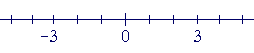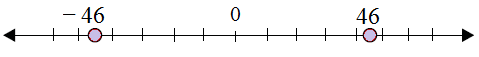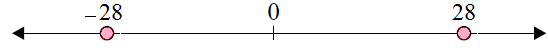# Plotting opposite integers on a number line

The opposite of any number

Every number has an opposite number. The opposite of integer 3, for example, is found at the same distance from 0, but on the other side as −3.Similarly, the negative of integer −3 is at the same distance from 0, but on the other side and it is +3.

−(−3) = 3.

So, the opposite of integer −3 is 3.

This is true for any integer ‘a’:

−(−a) = a

The opposite of −a is a.

For example,

−(−12) = 12.

Rules for plotting opposite integers on a number line

• For any given integer ‘a’, the opposite integer is found at the same distance from 0 on the number line, but on the other side.

• So, the opposite integer of integer ‘a’, is ‘-a’ on the number line, but on the other side of 0;

• So, the opposite integer of integer ‘-a’, is ‘a’ on the number line, but on the other side of 0.

Plot the integer −46 and its opposite on the number line.

### Solution

Step 1:

The opposite of −46 is 46.

Step 2:

These integers on number line are as shown below.Plot the integer 28 and its opposite on the number line.

### Solution

Step 1:

The opposite of 28 is −28.

Step 2:

These integers on number line are as shown below.